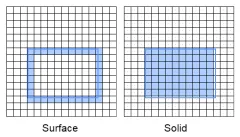# Procedural generation optimization (hollow terrain)

I need to optimize my terrain but I don’t know how to

(outside)

(inside)

I have tried doing a i,v pairs loop but it was laggy and didnt work.

This is my code V

``````local replicatedStorage = game:GetService("ReplicatedStorage")
local runService = game:GetService("RunService")

local partContainer = Instance.new("Folder",workspace) partContainer.Name = "PerlinParts"
local propContainer = workspace:FindFirstChild("Props")

local blockSize = 2

local mapxSize = 16
local mapySize = 64
local mapzSize = 64

local noiseScale = 50
local amplitude = 12

local seed = math.random(300,5000000)

local function GenerateNoiseMap()
for x = 0,mapxSize do
for y=-mapySize/2, mapySize/2 do
for z = 0,mapzSize do
local xn = math.noise(y/noiseScale, z/noiseScale, seed) * amplitude
local yn = math.noise(x/noiseScale, z/noiseScale, seed) * amplitude
local zn = math.noise(x/noiseScale, y/noiseScale, seed) * amplitude
local density = xn + yn + zn + y

local part = Instance.new("Part")

if density <= 25 then
part = Instance.new("Part",partContainer) part.Anchored = true part.Size = Vector3.new(blockSize,blockSize,blockSize)
part.CFrame = CFrame.new(x*blockSize, y*blockSize, z*blockSize)
part.TopSurface = "Smooth" part.BottomSurface = "Smooth"
elseif density <= 24 then
return density
end

if density >= 25 then
local colorGenerator = math.random(90,100)
part.Color = Color3.fromRGB(colorGenerator,colorGenerator,colorGenerator)
end

if density <= 25 and density > 22 then
part.Color = Color3.fromRGB(math.random(90,110),math.random(160,180),math.random(0,10))
end
if density <= 22 and density > 17 then
part.Color = Color3.fromRGB(math.random(114,134),math.random(82,102),math.random(60,80))
end
if density <= 17 and density > 10 then
local colorGenerator = math.random(111,121)
part.Color = Color3.fromRGB(colorGenerator,colorGenerator,colorGenerator)
end
if density <= 10 then
local colorGenerator = math.random(90,100)
part.Color = Color3.fromRGB(colorGenerator,colorGenerator,colorGenerator)
end

end
runService.Heartbeat:Wait()
end
end
end

GenerateNoiseMap()
``````

you can reduce the amount of if statements

That doesnt do anything ㅤㅤㅤㅤㅤㅤㅤㅤㅤㅤㅤㅤㅤㅤㅤ

you could look into Greedy Meshers for lower part counts

the main optimisation you need to do is culling

you first need to loop and save the block data in a table

then you loop again but this time you only create parts that are touching air

any block that is surrounded by 6 surrounding blocks should be skipped as you cant see them anyway

so the end result will look like the image on the left sideits also possible to do greedy mesh but its not mandatory

here is a video i made showing how to do greedy mesh

1 Like